In the last yarlp blog post, I ran Double Deep Q-Learning on Atari, which took around 1-1.5 days to train per Atari environment for 40M frames. I wanted to implement something faster, namely A2C (Advantage Actor Critic). It’s related to A3C by Mnih et al, 2016, without the asynchronous part.

A2C works like so:

1. Create $N_e$ (16) environments that will execute in parallel.
2. Execute rollouts for $N_s$ (5) time-steps in each environment.
3. Calculate the discounted $N_s$-step returns, which we call $R$.
4. Calculate the advantage with $A(s) = V(s) - R$ where $V(s)$ is a function that takes states as inputs and outputs a prediction for $R$
5. Update a deep neural network according to the following loss: $\sum (R - V(s))^2 - log(\pi(s)) A(s)$, where $\pi(s)$ is the policy network that shares weights with $V(s)$ but outputs actions.
6. Repeat

A2C is sample inefficient, meaning it doesn’t learn as quickly per frame compared to DDQN. But A2C can train on 40M frames within a couple of hours with 16 threads compared to 1-1.5 days, solving environments like Pong and Breakout a lot faster. The learning curves by frames (left) and by wall-clock time (right) are shown below.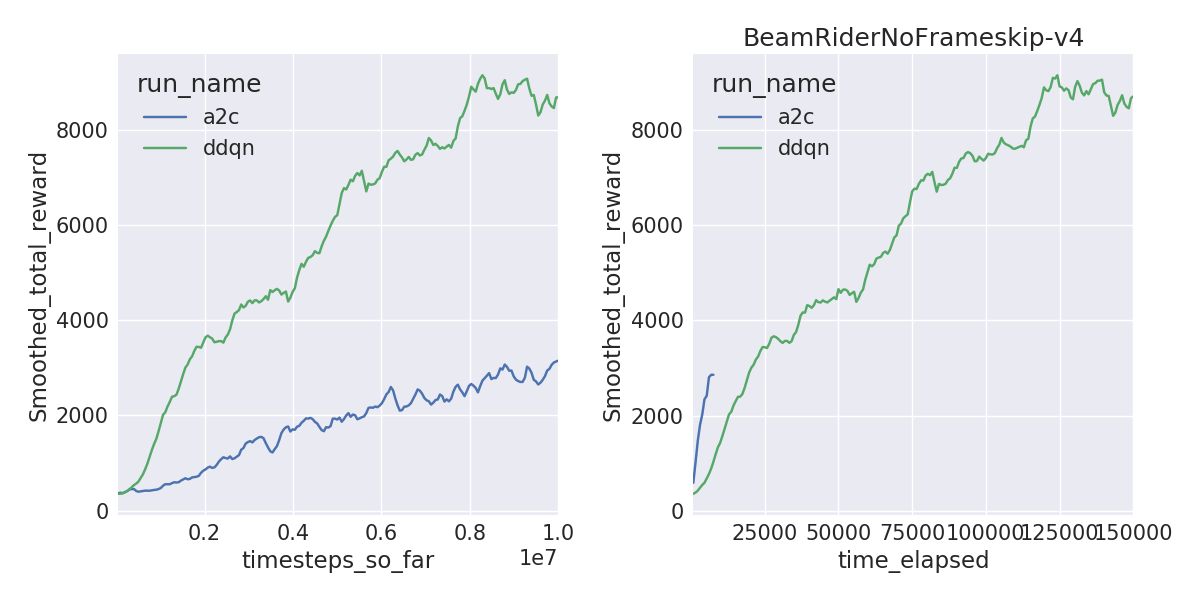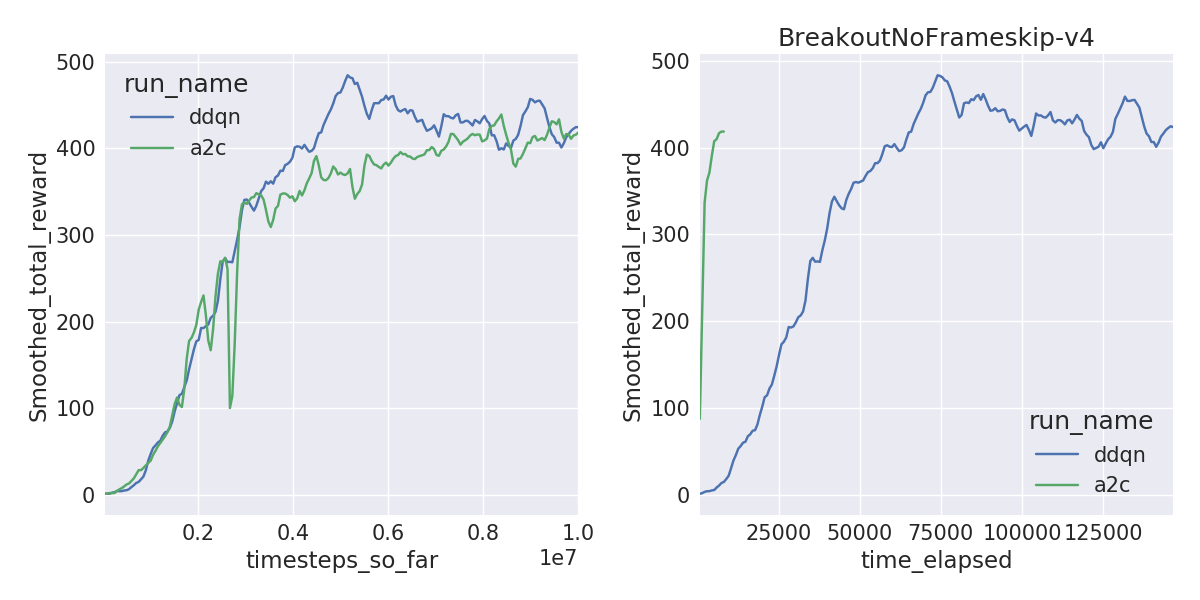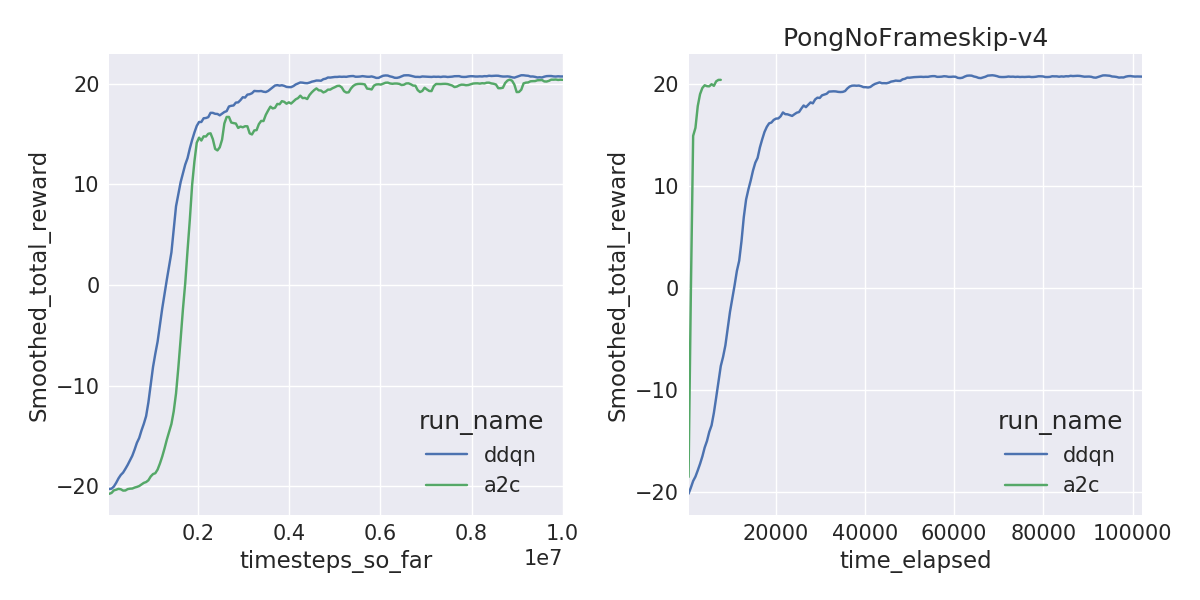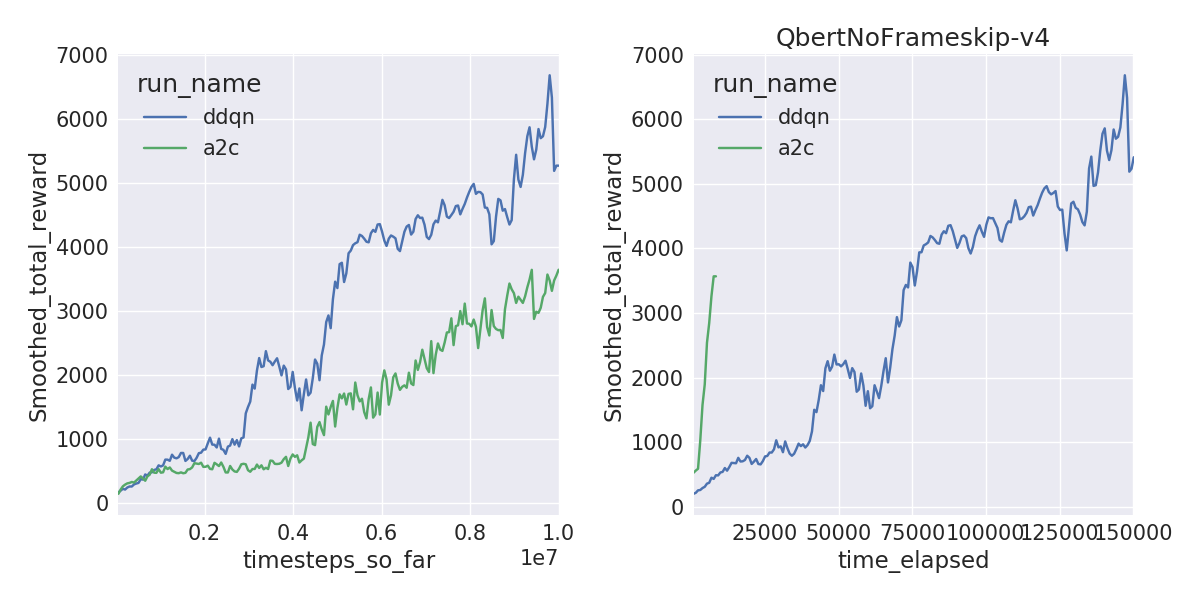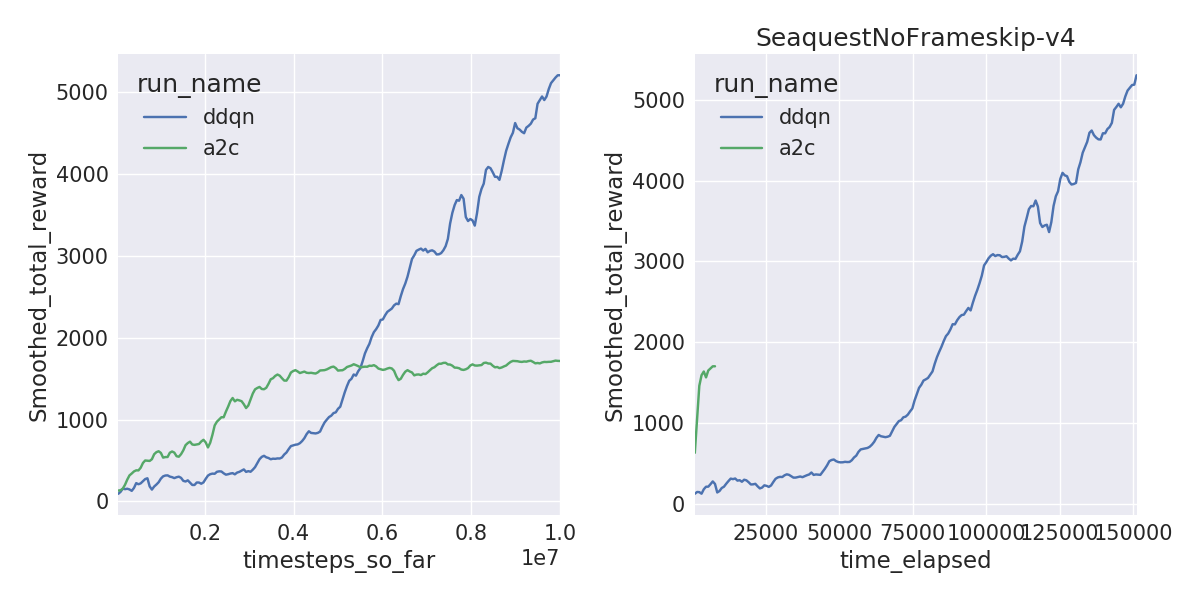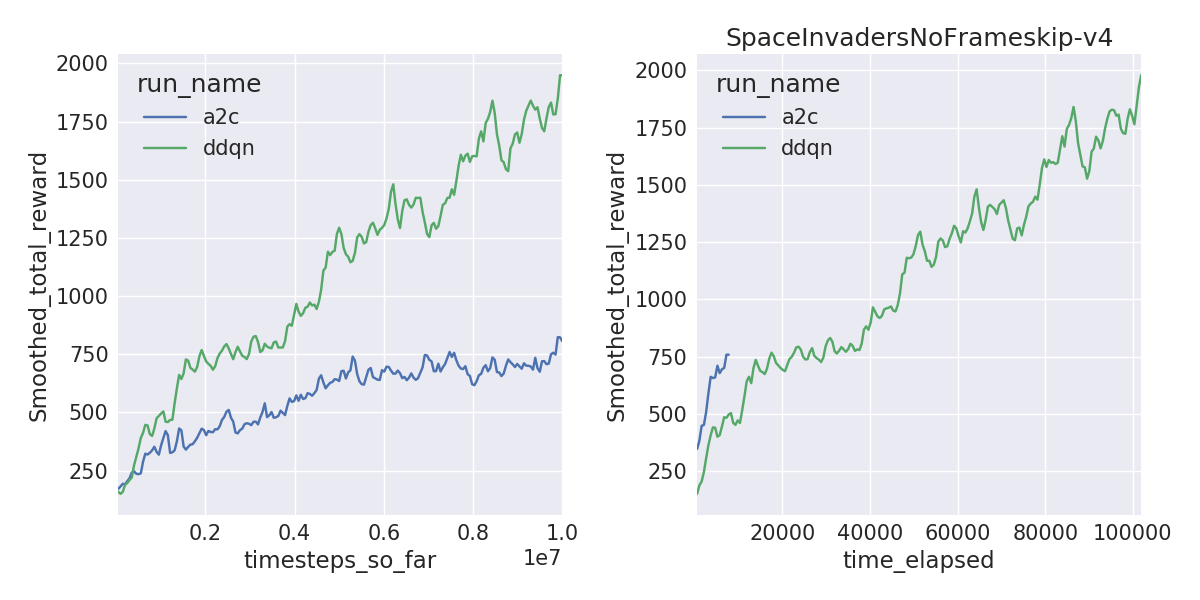I added an implementation of A2C to yarlp.

You may be thinking, why not add experience replay to A2C to make it more sample efficient? And while we’re at it, why not add some trust-region update as well? I think this turns out to be ACER, which I don’t think I’ll implement (it has a lot of moving parts).

I have recently read and really appreciate Ben Recht’s posts on An Outsider’s Tour of of Reinforcement Learning. Recht’s students recently published a paper showing that random search with linear policies can perform as well as, if not better than TRPO. I hope RL algos stay simple and become more stable!